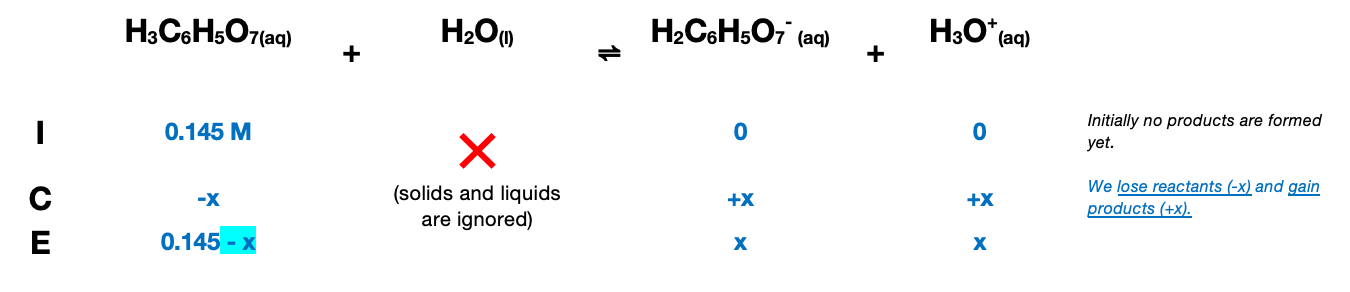# Problem: Calculate the [H3O+] of the following polyprotic acid solution: 0.145M H3C6H5O7.Calculate the pH of this solution.

###### FREE Expert Solution

Since we’re dealing with a weak acid, we will have to create an ICE chart to determine the equilibrium concentration of each species:$\overline{){\mathbf{K}}{\mathbf{=}}\frac{\mathbf{p}\mathbf{r}\mathbf{o}\mathbf{d}\mathbf{u}\mathbf{c}\mathbf{t}\mathbf{s}}{\mathbf{r}\mathbf{e}\mathbf{a}\mathbf{c}\mathbf{t}\mathbf{a}\mathbf{n}\mathbf{t}\mathbf{s}}}$

Solids and liquids are not included in the expression

${\mathbit{K}}_{\mathbf{1}}\mathbf{=}\frac{\left[{\mathbf{H}}_{\mathbf{2}}{\mathbf{C}}_{\mathbf{6}}{\mathbf{H}}_{\mathbf{5}}{{\mathbf{O}}_{\mathbf{7}}}^{\mathbf{-}}\right]\left[{\mathbf{H}}_{\mathbf{3}}{\mathbf{O}}^{\mathbf{+}}\right]}{\left[{\mathbf{H}}_{\mathbf{3}}{\mathbf{C}}_{\mathbf{6}}{\mathbf{H}}_{\mathbf{5}}{\mathbf{O}}_{\mathbf{7}}\right]}\phantom{\rule{0ex}{0ex}}\mathbf{8}\mathbf{.}\mathbf{4}\mathbf{×}{\mathbf{10}}^{\mathbf{-}\mathbf{4}}\mathbf{=}\frac{\left(\mathbf{x}\right)\left(\mathbf{x}\right)}{\left(\mathbf{0}\mathbf{.}\mathbf{145}\mathbf{-}\mathbf{x}\right)}\phantom{\rule{0ex}{0ex}}\mathbf{8}\mathbf{.}\mathbf{4}\mathbf{×}{\mathbf{10}}^{\mathbf{-}\mathbf{4}}\mathbf{=}\frac{{\mathbf{x}}^{\mathbf{2}}}{\mathbf{\left(}\mathbf{0}\mathbf{.}\mathbf{145}\mathbf{-}\mathbf{x}\mathbf{\right)}}$

Check if the x in the denominator can be ignored

since 172 < 500, x in the denominator cannot be ignored

$\left\{8.4×{10}^{-4}=\frac{{x}^{2}}{\overline{)\left(0.145-x\right)}}\right\}\overline{)\mathbf{\left(}\mathbf{0}\mathbf{.}\mathbf{145}\mathbf{-}\mathbf{x}\mathbf{\right)}}\phantom{\rule{0ex}{0ex}}\phantom{\rule{0ex}{0ex}}\left(8.4×{10}^{-4}\right)\left(0.145-x\right){\mathbf{=}}{{\mathbf{x}}}^{{\mathbf{2}}}\phantom{\rule{0ex}{0ex}}\phantom{\rule{0ex}{0ex}}{\mathbf{1}}{\mathbf{.}}{\mathbf{218}}{\mathbf{×}}{{\mathbf{10}}}^{\mathbf{-}\mathbf{4}}{\mathbf{-}}\mathbf{\left(}\mathbf{8}\mathbf{.}\mathbf{4}\mathbf{×}{\mathbf{10}}^{\mathbf{-}\mathbf{4}}\mathbf{\right)}{\mathbf{x}}{\mathbf{=}}{{\mathbf{x}}}^{{\mathbf{2}}}\phantom{\rule{0ex}{0ex}}\phantom{\rule{0ex}{0ex}}{{\mathbf{x}}}^{{\mathbf{2}}}{\mathbf{+}}\mathbf{\left(}\mathbf{8}\mathbf{.}\mathbf{4}\mathbf{×}{\mathbf{10}}^{\mathbf{-}\mathbf{4}}\mathbf{\right)}{\mathbf{x}}{\mathbf{-}}{\mathbf{1}}{\mathbf{.}}{\mathbf{218}}{\mathbf{×}}{{\mathbf{10}}}^{\mathbf{-}\mathbf{4}}{\mathbf{=}}{\mathbf{0}}$

Solve for x using the quadratic formula:

98% (444 ratings)###### Problem Details

Calculate the [H3O+] of the following polyprotic acid solution: 0.145M H3C6H5O7.

Calculate the pH of this solution.